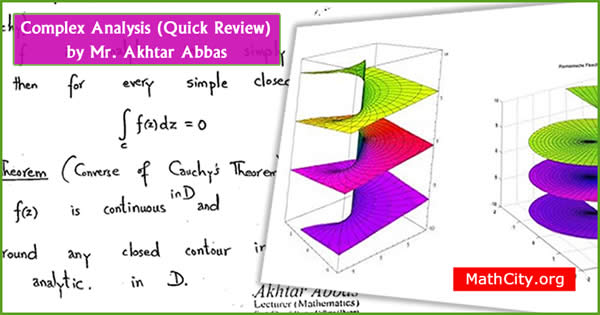# Complex Analysis (Quick Review)These notes are made and shared by Mr. Akhtar Abbas. We are really very thankful to him for providing these notes and appreciates his efforts to publish these notes on MathCity.org. Important definitions and important results are the part of these notes, these might be helpful to prepare interviews or any other written test after graduation like PPSC, FPSC or etc.

 Name Complex Analysis (Quick Review) Mr. Akhtar Abbas 24 pages PDF (see Software section for PDF Reader) 385 kB
• If any pair of points $z_1, z_2 \in S$ can be connected by a polygonal line that consists of a finite number of line segments joined end to end that lies entirely in the set, then $S$ is connected.
• A function $v(x,y)$ is harmonic conjugate of $u(x,y)$ if $f(z)=u(x,y)+iv(x,y)$ is analytics.
• Theorem (Cauchy): If $f$ is analytic in simply connected domain $D$, then for every simple closed contour $C$ in $D$, $\int_C f(z) dz =0$.
• A number $z_0$ is zero of $f(z)$ if $f(z_0)=0$.

Please click on View Online to see inside the PDF.

• notes/complex-analysis-quick-review